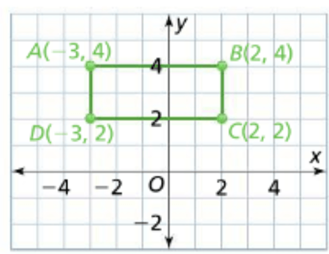Mathematics
Easy

Question

# Calculate the area of rectangle ABCD.## 10 sq. units    14 sq. units    12 sq. units    8 sq. unitsHint:

## The correct answer is: 10 sq. units

### The coordinates of the rectangle ABCD areA is (-3,4)B is (2,4)C is (2,2)D is (-3,2)We have to find the length of the adjacent sides.To find the length we will use the following criteria:1) If x coordinate is same, we will find the absolute distance using y coordinate. An absolute distance is the length of the point from the respective axis. If x coordinate is same then its length is measured from x axis. It is a positive quantity.2) If y coordinate is same we will use the x coordinate to find absolute distance.3) As the points are in adjacent quadrants, we will have to add the absolute distances to find length.We will consider the side AB as length and BC as breadthWe will find the length of side AB.For the points A and B, y coordinate is same. We will find the absolute distance using x coordinate.Absolute distance of the point A = |-3|Absolute distance of the point B = |2|Length of AB = |-3| + |2|= 3 + 2= 5Length of the rectangle is 5 units.We will find the length of side BC.For the points B and C, x coordinate is same. We will find the absolute distance using x coordinate.Absolute distance of the point B = |4|Absolute distance of the point C = |2|Length of BC = |4| - |2|= 4 - 2= 2Breadth of the rectangle is 2 units.The formula for perimeter is (length × breadth)Perimeter of the rectangle ABCD = (length × breadth)= (5 × 2)= 10 sq. unitsTherefore, perimeter of the rectangle is 10 sq. units.

For such questions, we have to see if the points of the rectangle are in same quadrant or adjacent quadrants. For points in same quadrants, we have to subtract the absolute values. We can use distance formula to find the length of sides of rectangle too.

### Related Questions to study#### With Turito Foundation.#### Get an Expert Advice From Turito.